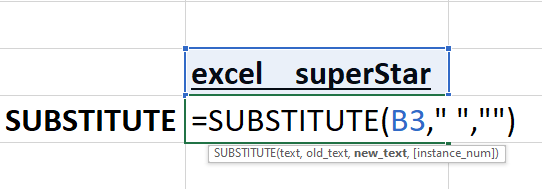# Excel Substitute FormulaHello Everyone, In this tutorial, we will be learning Substitute Formula to Replace in Excel.Assume that you have a text written in a cell, and you want no spacing in that cell. So to make your cell look beautiful, we will be applying the SUBSTITUTE Formula in Excel by following the steps given below:

1. Press =SUBSTITUTE, and open the bracket =SUBSTITUTE(Now there are 3 main parameters in the formula (text, old_text, new_test) The First parameter text means to choose the cell which has text inside it

2. Choose the cell which has text inside it and press comma(,) =SUBSTITUTE(B3,

The second parameter old_text means the text which is old and you want to remove it from the cell

3. Press Double quotation and put space and again press Double quotation =SUBSTITUTE(B3, “ ”,

Note – Press Comma after every parameter

The Third Parameter new_text means putting spaces in Double quotation which substitute you want to put in

4. Press Double quotation two times and close the bracket =SUBSTITUTE (B3, “ ”,””)

5. Lastly press Enter and your characters will be replaced using SUBSTITUTE Formula.